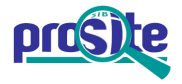Entry: PS00265

 Entry name [info] PANCREATIC_HORMONE_1 Accession [info] PS00265 Entry type [info] PATTERN Date [info] 01-APR-1990 CREATED; 01-NOV-1995 DATA UPDATE; 02-JUN-2021 INFO UPDATE. PROSITE Doc. [info] PDOC00238

Name and characterization of the entry

 Description [info] Pancreatic hormone family signature. Pattern [info] ```[FY]-x(3)-[LIVM]-x(2)-Y-x(3)-[LIVMFY]-x-R-x-R-[YF]. ```

Numerical results [info]

Numerical results for UniProtKB/Swiss-Prot release 2021_03 which contains 565'254 sequence entries.

 Total number of hits 89 in 89 different sequences Number of true positive hits 86 in 86 different sequences Number of 'unknown' hits 0 Number of false positive hits 3 in 3 different sequences Number of false negative sequences 7 Number of 'partial' sequences 7 Precision (true positives / (true positives + false positives)) 96.63 % Recall (true positives / (true positives + false negatives)) 92.47 %

 UniProtKB/Swiss-ProtTrue positive sequences 86 sequences ```NPF_ARTTR (P41334 ), NPF_CORAP (P41321 ), NPF_LOCMI (P86442 ), ``` ```NPF_MONEX (P41967 ), NPY1_CONBE (P0CJ22 ), NPY2_CONBE (P0CJ23 ), NPYV_MEIAT (P0DP55 ), NPY_ALLMI (P68007 ), NPY_APLCA (Q27441 ), NPY_BOVIN (Q6RUW3 ), NPY_CARAU (P28672 ), NPY_CAVPO (P68008 ), NPY_CHICK (P28673 ), NPY_CYPCA (Q9DGK7 ), NPY_DANRE (Q9I8P3 ), NPY_DICLA (Q9PTA0 ), NPY_GADMO (P80167 ), NPY_HUMAN (P01303 ), NPY_ICTPU (Q9I9D3 ), NPY_LAMFL (P48097 ), NPY_MACMU (Q9XSW6 ), NPY_MOUSE (P57774 ), NPY_ONCMY (P29071 ), NPY_PAROL (Q90WF4 ), NPY_PELRI (P69101 ), NPY_PIG (P01304 ), NPY_RABIT (P68006 ), NPY_RANTE (P69102 ), NPY_RAT (P07808 ), NPY_SHEEP (P14765 ), NPY_TORMA (P28674 ), NPY_TYPNA (Q9PW68 ), NPY_XENLA (P33689 ), PAHO_ALLMI (P06305 ), PAHO_ANSAN (P06304 ), PAHO_BOVIN (P01302 ), PAHO_CANLF (P01299 ), PAHO_CAVPO (P13083 ), PAHO_CERSI (P37999 ), PAHO_CHICH (P41519 ), PAHO_CHICK (P68248 ), PAHO_DIDVI (P18107 ), PAHO_EQUPR (P68010 ), PAHO_EQUZE (P68009 ), PAHO_ERIEU (P41335 ), PAHO_FELCA (P06884 ), PAHO_HUMAN (P01298 ), PAHO_LARAR (P41337 ), PAHO_LITCT (P15427 ), PAHO_MACMU (P33684 ), PAHO_MELGA (P68249 ), PAHO_MOUSE (P10601 ), PAHO_PIG (P01300 ), PAHO_RABIT (P41336 ), PAHO_RANTE (P31229 ), PAHO_RAT (P06303 ), PAHO_SHEEP (P01301 ), PAHO_STRCA (P11967 ), PAHO_TAPPI (P39659 ), PMY_PETMA (P80024 ), PPY_LOPAM (P09475 ), PYYA_DANRE (Q9I8P2 ), PYYV_VARER (E2E4L2 ), PYY_AMICA (P29205 ), PYY_ANGJA (Q76CL2 ), PYY_ATRSP (P69094 ), PYY_BERRH (P29206 ), PYY_BOVIN (P51694 ), PYY_CANLF (P68004 ), PYY_CHICK (P29203 ), PYY_DICLA (Q9PT99 ), PYY_HUMAN (P10082 ), PYY_LAMFL (P48098 ), PYY_MOUSE (Q9EPS2 ), PYY_MYOSC (P09641 ), PYY_ONCKI (P69092 ), PYY_ONCMY (P69093 ), PYY_ORENI (P81028 ), PYY_PELRI (P29204 ), PYY_PIG (P68005 ), PYY_RABIT (Q9TR93 ), PYY_RAT (P10631 ), PYY_SCYCA (P69095 ), PYY_SQUAC (P69096 ), PY_DICLA (Q9PT98 ), SPYY_PHYBI (P80952 ) ``` » more Retrieve an alignment of UniProtKB/Swiss-Prot true positive hits: [Clustal format, color, condensed view] [Clustal format, color] [Clustal format, plain text] [Fasta format] Retrieve the sequence logo from the alignment UniProtKB/Swiss-ProtFalse negative sequences 7 sequences ```NPF_AEDAE (Q8MP00 ), NPF_ANOGA (Q7Q7R8 ), NPF_DROME (Q9VET0 ), ``` ```NPF_DROPS (Q29B55 ), PYY2_BOVIN (P06833 ), PYY2_HUMAN (Q9NRI6 ), PYY3_HUMAN (Q5JQD4 ) ``` » more UniProtKB/Swiss-Prot'Partial' sequences 7 sequences ```NPF_HELZE (P85106 ), NPF_SCHGR (P86443 ), PYF1_PENMO (P84005 ), ``` ```PYF2_PENMO (P84006 ), PYF3_PENMO (P84007 ), PYF4_PENMO (P84008 ), PYF_LOLVU (P84004 ) ``` » more UniProtKB/Swiss-ProtFalse positive sequences 3 sequences ```FAT1_YEAST (P38225 ), M17L2_DANRE (Q6DGV7 ), XTH27_ARATH (Q8LDS2 ) ``` PDB[Detailed view] 21 PDB 1BBA; 1F8P; 1K8V; 1LJV; 1PPT; 1QBF; 1RON; 1RU5; 1RUU; 1TZ4; 1TZ5; 2DEZ; » more